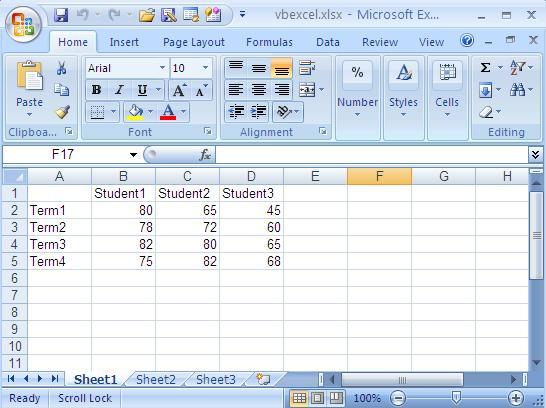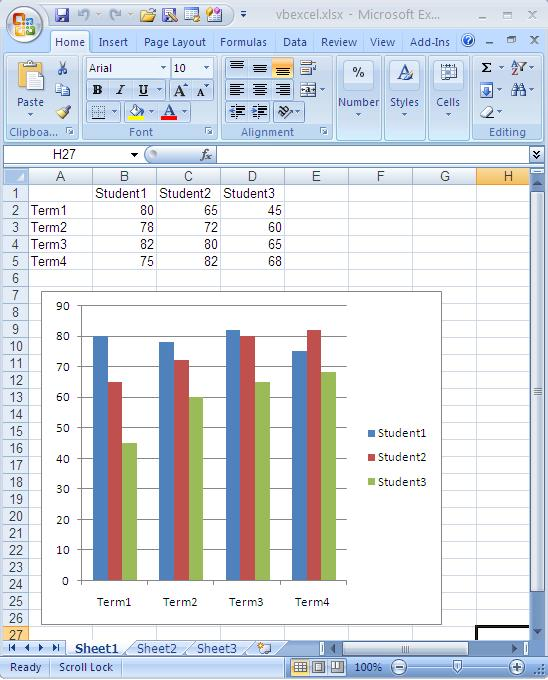VB.Net Tutorial

# Excel Chart in VB.NET

We have the ability to generate dynamic and visually appealing charts in Excel by leveraging the capabilities of VB.NET. In the following section, we will explore the process of creating a chart in Excel through VB.NET source code, enabling us to effectively represent data in a graphical format.

Before we proceed with creating a chart, it is essential to populate the Excel sheet with the relevant data. Once the data has been entered, the Excel sheet will begin to take shape, serving as the foundation for our chart creation. This step ensures that the data is organized and structured appropriately for chart generation.

To initiate the chart creation process in VB.NET, we must first create a chart object. This object acts as a container for our chart and allows us to configure various aspects such as its position, size, data range, and chart type. By customizing these parameters, we can tailor the chart to suit our specific requirements and visual preferences.It is crucial to carefully select the appropriate chart type that best represents the underlying data effectively. Different chart types, such as bar charts, line charts, pie charts, and scatter plots, offer unique visual representations and insights. By understanding the nature of the data and the desired analytical goals, we can make an informed decision when choosing the chart type.

Once the chart object is created and configured, we can populate it with the necessary data range from the Excel sheet. This ensures that the chart accurately reflects the underlying data and provides a clear visual representation. By defining the data range, we establish the connection between the Excel sheet and the chart, allowing for real-time updates as the data changes.

The following picture shows the excel file after created a chart.Through the seamless integration of VB.NET and Excel, we gain the ability to create powerful and visually compelling charts that enhance data analysis and presentation. This combination of programming capabilities and Excel's charting functionalities empowers us to deliver insightful and impactful visualizations, facilitating effective communication and decision-making processes.

Full Source VB.NET
Imports Excel = Microsoft.Office.Interop.Excel Public Class Form1 Private Sub Button1_Click(ByVal sender As System.Object, _ ByVal e As System.EventArgs) Handles Button1.Click Dim xlApp As Excel.Application Dim xlWorkBook As Excel.Workbook Dim xlWorkSheet As Excel.Worksheet Dim misValue As Object = System.Reflection.Missing.Value xlApp = New Excel.ApplicationClass xlWorkBook = xlApp.Workbooks.Add(misValue) xlWorkSheet = xlWorkBook.Sheets("sheet1") 'add data xlWorkSheet.Cells(1, 1) = "" xlWorkSheet.Cells(1, 2) = "Student1" xlWorkSheet.Cells(1, 3) = "Student2" xlWorkSheet.Cells(1, 4) = "Student3" xlWorkSheet.Cells(2, 1) = "Term1" xlWorkSheet.Cells(2, 2) = "80" xlWorkSheet.Cells(2, 3) = "65" xlWorkSheet.Cells(2, 4) = "45" xlWorkSheet.Cells(3, 1) = "Term2" xlWorkSheet.Cells(3, 2) = "78" xlWorkSheet.Cells(3, 3) = "72" xlWorkSheet.Cells(3, 4) = "60" xlWorkSheet.Cells(4, 1) = "Term3" xlWorkSheet.Cells(4, 2) = "82" xlWorkSheet.Cells(4, 3) = "80" xlWorkSheet.Cells(4, 4) = "65" xlWorkSheet.Cells(5, 1) = "Term4" xlWorkSheet.Cells(5, 2) = "75" xlWorkSheet.Cells(5, 3) = "82" xlWorkSheet.Cells(5, 4) = "68" 'create chart Dim chartPage As Excel.Chart Dim xlCharts As Excel.ChartObjects Dim myChart As Excel.ChartObject Dim chartRange As Excel.Range xlCharts = xlWorkSheet.ChartObjects myChart = xlCharts.Add(10, 80, 300, 250) chartPage = myChart.Chart chartRange = xlWorkSheet.Range("A1", "d5") chartPage.SetSourceData(Source:=chartRange) chartPage.ChartType = Excel.XlChartType.xlColumnClustered xlWorkSheet.SaveAs("C:\vbexcel.xlsx") xlWorkBook.Close() xlApp.Quit() releaseObject(xlApp) releaseObject(xlWorkBook) releaseObject(xlWorkSheet) MsgBox("Excel file created , you can find the file c:\") End Sub Private Sub releaseObject(ByVal obj As Object) Try System.Runtime.InteropServices.Marshal.ReleaseComObject(obj) obj = Nothing Catch ex As Exception obj = Nothing Finally GC.Collect() End Try End Sub End Class

### Conclusion

Following the outlined steps and utilizing the provided VB.NET source code, we can unlock the potential of Excel's charting capabilities and create professional-quality charts that add value to our data-driven endeavors.